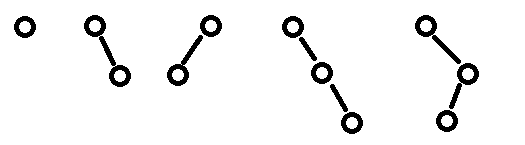/ Vijos / 题库 /

# 描述

—— 左子树序号大于L
—— 左子树序号等于L且右子树序号大于R# 样例1

## 样例输入1

``````43
3254
233212
0
``````

## 样例输出1

``````(X(X))X((X)X)
X((((X)X)X((X(X(X)))X))X)
(X(X((X)X(X((X(X))X(X(X(X))))))))X
``````

## 样例输入2

``````1
2
3
4
5
0
``````

## 样例输出2

``````X
X(X)
(X)X
X(X(X))
X((X)X)
``````

# 限制

ONLY 1S，可怜的cpc。。。。

ID
1400

2

(无)

176

96

55%